﻿ 基于双边核估计的保持跳跃曲线回归过程 A Jump-Preserving Curve Regression Procedure Based on Bilateral Kernel Estimation

Statistical and Application
Vol.04 No.04(2015), Article ID:16725,13 pages
10.12677/SA.2015.44037

A Jump-Preserving Curve Regression Procedure Based on Bilateral Kernel Estimation

Yiran Li, Xingfang Huang*, Jiazhao Ding, Xuan Chen

Department of Mathematics, Southeast University, Nanjing Jiangsu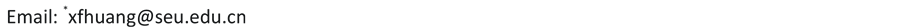Received: Dec. 10th, 2015; accepted: Dec. 28th, 2015; published: Dec. 31st, 2015ABSTRACT

It is well known that curve regression is very important in many applications. However, since data collection procedures are disturbed by errors, traditional curve regression methods cannot play well in jump points. This paper proposes a jump-preserving curve fitting procedure, which is based on bilateral kernel estimation. Kernel functions are not only added to x-axis, but also added to y-axis. Then, we estimate given points from left side, right side and whole neighborhood. Weighted residual sums of squares are calculated to compare. The estimate with smaller weighted residual sums of squares is selected as the final estimate of the given point, so that we can achieve jump- preserving while not to detect jump points at first. Numerical simulation and real data analysis demonstrate the feasibility and efficiency of this method.

Keywords:Curve Fitting Procedure, Jump-Preserving, Bilateral Kernel Estimation, Weighted Residual Sums of Squares, Discontinuous Curve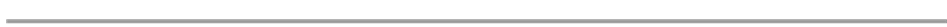1. 引言

2. 跳跃回归模型(1)(2)

3. 基于双边核的保跳曲线拟合过程(3)

1)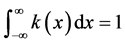2)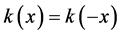(4)(5)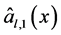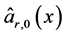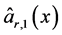(6)(7)(8)(9)(10)(11)(12)(13)(14)(15)(16)(17)(18)(19)， (20)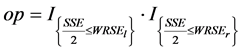, (21)为示性函数，下标条件成立时取值为1；否则为0。(22)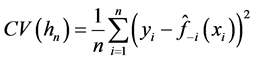(23)

4. 数值实验

4.1. 仿真模拟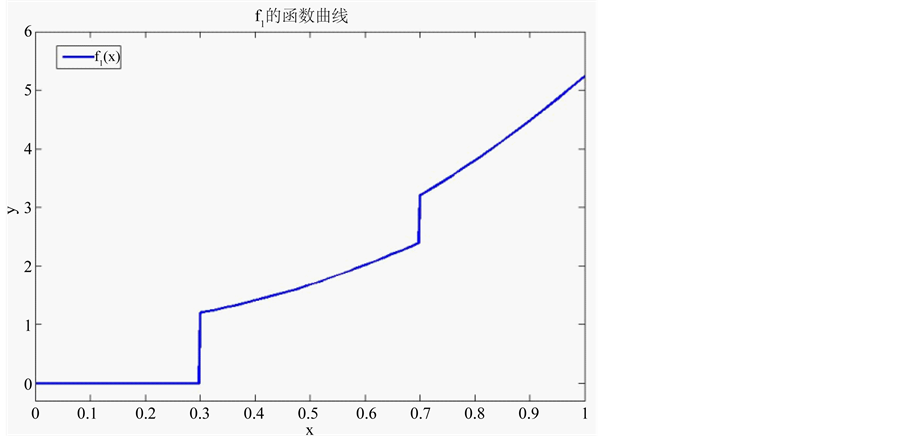Figure 1. Original image of f1(x)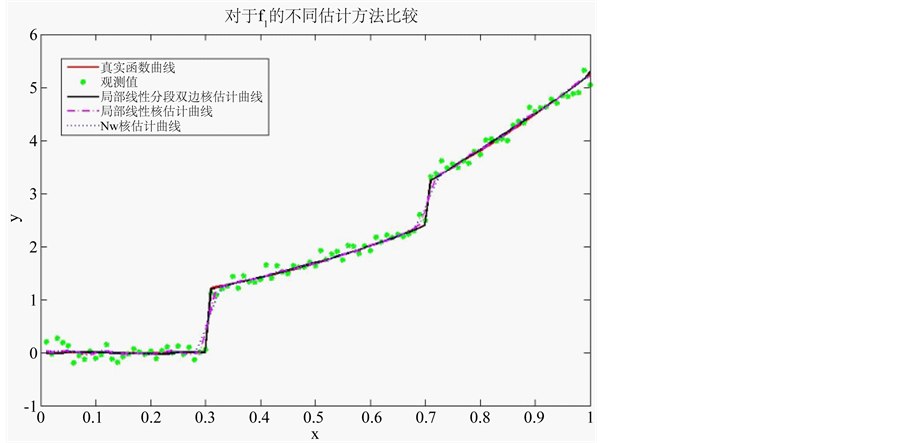Figure 2. Comparison among different estimation methods of f1(x)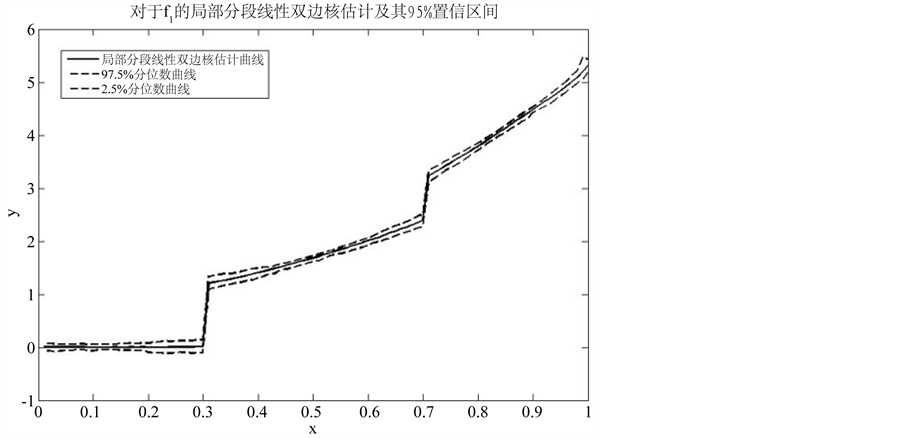Figure 3. Confidence interval of f1(x)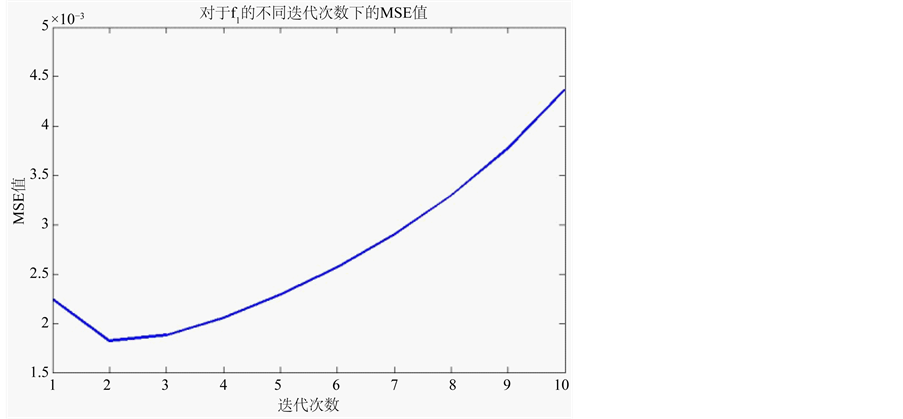Figure 4. f1(x) MSE of local piecewise linear bilateral Kernel estimation under different iteration timesTable 1. MSE value for different estimation method of f1(x)4.2. 数值实例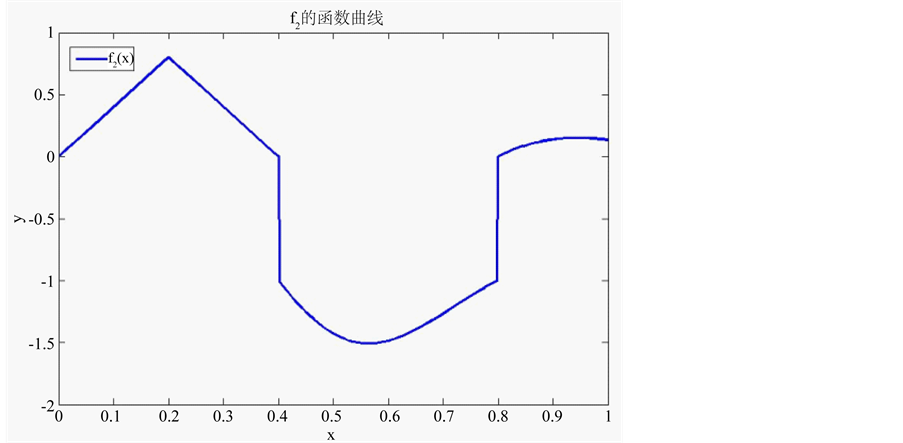Figure 5. Original image of f2(x)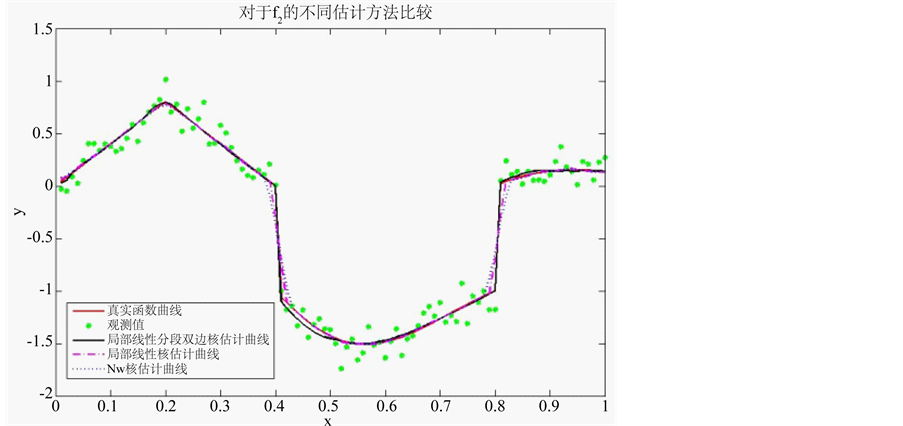Figure 6. Comparison among different estimation methods of f2(x)Figure 7. Confidence Interval of f2(x)Table 2. MSE value for different estimation method of f2(x)Figure 8. f2(x) MSE of local piecewise linear bilateral Kernel estimation under different iteration times

5. 讨论

5.1. 光滑性

5.2. 带宽选择Figure 9. Real data image and its fitting result

5.3. 迭代次数

A Jump-Preserving Curve Regression Procedure Based on Bilateral Kernel Estimation[J]. 统计学与应用, 2015, 04(04): 335-347. http://dx.doi.org/10.12677/SA.2015.44037

1. 1. Müller, H.-G. (1992) Change-Points in Nonparametric Regression Analysis. The Annuals of Statistics, 20, 737-761. http://dx.doi.org/10.1214/aos/1176348654

2. 2. Qiu, P., Cho, A. and Li, X. (1991) Estimation of Jump Regression Functions. Bulletin of Information and Cybernetics, 24, 197-212.

3. 3. Wu, J.S. and Chu, C.K. (1993) Kernel Type Es-timators of Jump Points and Values of a Regression Function. The Annals of Statistics, 21, 1545-1566. http://dx.doi.org/10.1214/aos/1176349271

4. 4. Loader, C.R. (1996) Change Point Estimation Using Nonparametric Regression. The Annals of Statistics, 24, 1667- 1678. http://dx.doi.org/10.1214/aos/1032298290

5. 5. Qiu, P. and Yandell, B.(1998) A Local Polynomial Jump Detection Algorithm in Nonparametric Regression. Technometrics, 40, 141-152.

6. 6. Wang, Y. (1995) Jump and Sharp Cusp Detection by Wavelets. Biometrika, 82, 385-397. http://dx.doi.org/10.1093/biomet/82.2.385

7. 7. Eubank, R.L. and Speckman, P.L. (1994) Nonparametric Estimation of Functions with Jump Discontinuities. In: Carlstein, E., Müller, H.G. and Siegmund, D., Eds., IMS Lecture Notes, Vol. 23, Change-Point Problems, 130-144. http://dx.doi.org/10.1214/lnms/1215463119

8. 8. Koo, J.Y. (1997) Spline Estimation of Discontinuous Regression Functions. Journal of Computational and Graphical Statistics, 6, 266-284.

9. 9. Shiau, J.H., Wahba, G. and Johnson, D.R. (1986) Partial Spline Models for the Inclusion of Tropopause and Frontal Boundary Information in Otherwise Smooth Two- and Three-Dimensional Objective Analysis. Journal of Atmospheric and Oceanic Technology, 3, 714-725. http://dx.doi.org/10.1175/1520-0426(1986)003<0714:PSMFTI>2.0.CO;2

10. 10. McDonald, J.A. and Owen, A.B. (1986) Smoothing with Split Linear Fits. Technometrics, 28, 195-208. http://dx.doi.org/10.1080/00401706.1986.10488127

11. 11. Qiu, P. (2003) A Jump-Preserving Curve Fitting Proce-dure Based on Local Piecewise-Linear Kernel Estimation. Jour- nal of Nonparametric Statistics, 15, 437-453. http://dx.doi.org/10.1080/10485250310001595083

12. 12. Fan, J. and Gijbels, I. (1996) Local Polynomial Modeling and Its Applications. Chapman & Hall, London, 66.

13. 13. Wikipedia, Kernel (Statistics). https://en.wikipedia.org/wiki/Kernel_(statistics)

14. 14. Silverman, B.W. (1986) Density Estimation for Statistics and Data Analysis. Chapman & Hall, London, 39, 296-297. http://dx.doi.org/10.1007/978-1-4899-3324-9

15. 15. Nadaraya, E.A. (1964) On Estimating Regression. Theory of Probability & Its Applications, 9, 141-142. http://dx.doi.org/10.1137/1109020

16. 16. Qiu, P. (1994) Estimation of the Number of Jumps of the Jump Regression Functions. Communications in Statistics-Theory and Methods, 23, 2141-2155. http://dx.doi.org/10.1080/03610929408831378

17. 17. Finance.yahoo.com. CEA Weekly-Adjusted Close Price. http://finance.yahoo.com/q/hp?s=CEA+Historical+Prices

18. 18. Loader, C.R. (1999) Bandwidth Selection: Classical or Plug-In? Annals of Statistics, 27, 415-438. http://dx.doi.org/10.1214/aos/1018031201

19. NOTES

*通讯作者。